# Matches

George poured out of the box matches and composing them triangles and no match was left. Then he tries squares, hexagons and octagons and no match was left. How many matches must be at least in the box?

Correct result:

n =  24

#### Solution: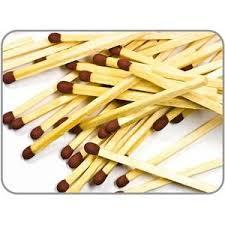We would be pleased if you find an error in the word problem, spelling mistakes, or inaccuracies and send it to us. Thank you!Tips to related online calculators
Do you want to calculate least common multiple two or more numbers?

#### You need to know the following knowledge to solve this word math problem:

We encourage you to watch this tutorial video on this math problem:

## Next similar math problems:

• Glass panelA rectangular glass panel with dimensions of 72 cm and 96 cm will cut the glazier on the largest square possible. What is the length of the side of each square? How many squares does the glazier cut?
• Dining tables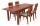In the dining room are tables with 4 chairs, 6 chairs, 8 chairs. How many diners must be at least to be occupy all tables (chairs) and diners are more than 50?
• PlumberPlumber had to cut the metal strip with dimensions 380 cm and 60 cm to the largest squares so that no waste. Calculate the length of the sides of a square. How many squares cut it?
• Endless lego setThe endless lego set contains only 6, 9, 20 kilograms blocks that can no longer be polished or broken. The workers took them to the gym and immediately started building different buildings. And of course, they wrote down how much the building weighed. The
• PexesoDrawing paper has dimensions of 60cm and 840mm. Pupils have to divide it into squares so that they can make a pexeso. What dimension must squares have if their side is to be larger than 3cm and less than 10cm?
• Largest squaresHow many of the largest square sheets did the plumber cut the honeycomb from 16 dm and 96 dm?
• Tractor wheelsThe front wheel of the tractor has a circumference of 18 dm and the rear 60 dm. We will make a red mark on the lowest point of both wheels. The tractor then starts. At what distance from the start will both marks appear identically at the bottom again?
• Around the flowerbed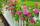Around a rectangular flowerbed with dimensions 5.25 m and 3.5 m are to be planted roses equally spaced so that the roses found in every corner of the flowerbed and consumed them as little as possible. a) At what distance are planted roses? b) How many ros
• Triangles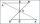Hanka cut the 20 cm long straws into three pieces each piece had a length in cm. Then, with these three pieces, she tried to make a triangle. a) What circuit has each of the triangles? b) How long can the longest side measure? c) How many different triang
• Lesson exercising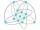The lesson of physical education, pupils are first divided into three groups so that each has the same number. The they redistributed, but into six groups. And again, it was the same number of children in each group. Finally they divided into nine equal g
• Gardens colonyGardens colony with dimensions of 180 m and 300 m are to be completely divided into the same large squares of the highest area. Calculate how many such squares can be obtained and determine the length of the square side.
• SchoolLess than 500 pupils attend school. When it is sorted into pairs, one pupil remains. Similarly, when sorted into 3, 4, 5 and 6 members team one remains. Sorted to seven members teams, no left behind. How many pupils are attending this school?
• TilesHall has dimensions 325 &time; 170 dm. What is the largest size of square tiles that can be entire hall tiled and how many we need them?
• Paper squaresThe paper rectangle measuring 69 cm and 46 cm should be cut into as many squares as possible. Calculate the lengths of squares and their number.
• Paving - jointsWe are paving with rectangular pavement 18 cm × 24 cm was placed side by side in height in a row and in the second row in width etc. How many times will the joints meet at a distance 10 m?
• Square roomWhat is the size of the smallest square room, which can pave with tiles with dimensions 55 cm and 45 cm? How many such tiles is needed?
• Z9–I–4 MO 2017Numbers 1, 2, 3, 4, 5, 6, 7, 8 and 9 were prepared for a train journey with three wagons. They wanted to sit out so that three numbers were seated in each carriage and the largest of each of the three was equal to the sum of the remaining two. The conduct